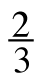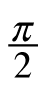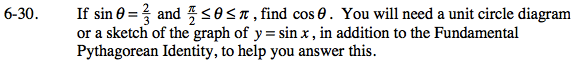### Home > PC > Chapter 6 > Lesson 6.1.2 > Problem6-30

6-30.If sin θ =andθπ, find cos θ. You will need a unit circle diagram or a sketch of the graph of y = sin x, in addition to the Fundamental Pythagorean Identity, to help you answer this. Homework Help ✎Draw a triangle diagram and label known sides.

Determine the length of the missing side.

Evaluate cos(θ). What sign should be used?